Скачать презентацию Bayes for Beginners Methods for Dummies FIL UCL

5aee6465a3c182a061b598141ad44731.ppt

• Количество слайдов: 68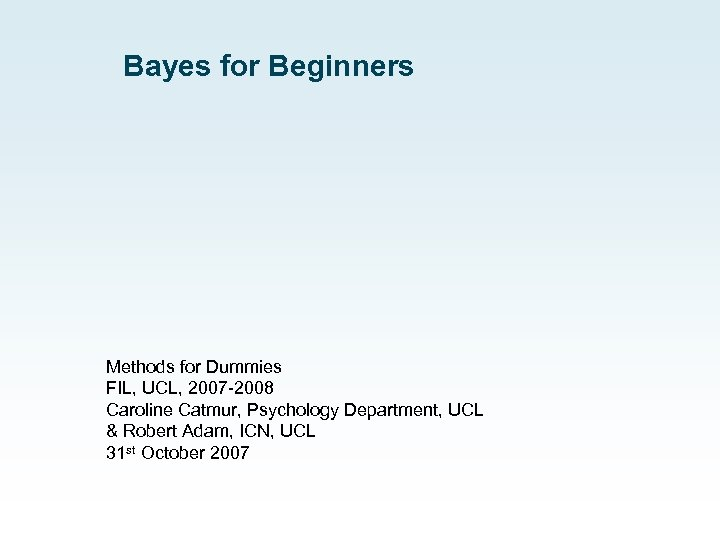Bayes for Beginners Methods for Dummies FIL, UCL, 2007 -2008 Caroline Catmur, Psychology Department, UCL & Robert Adam, ICN, UCL 31 st October 2007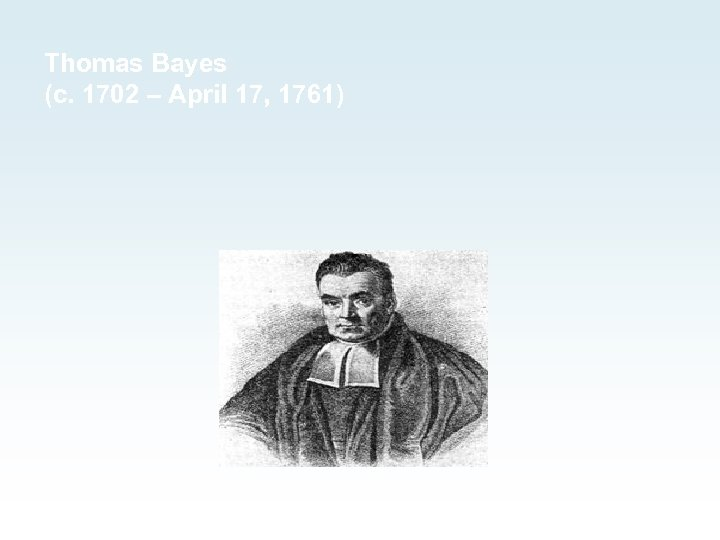Thomas Bayes (c. 1702 – April 17, 1761)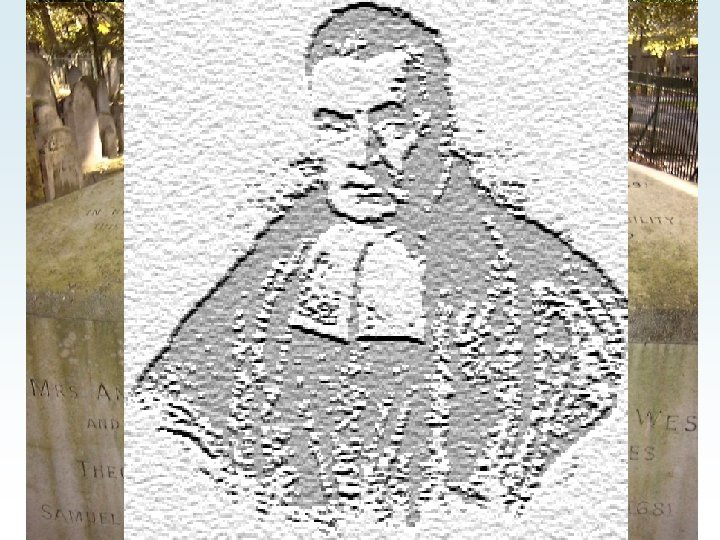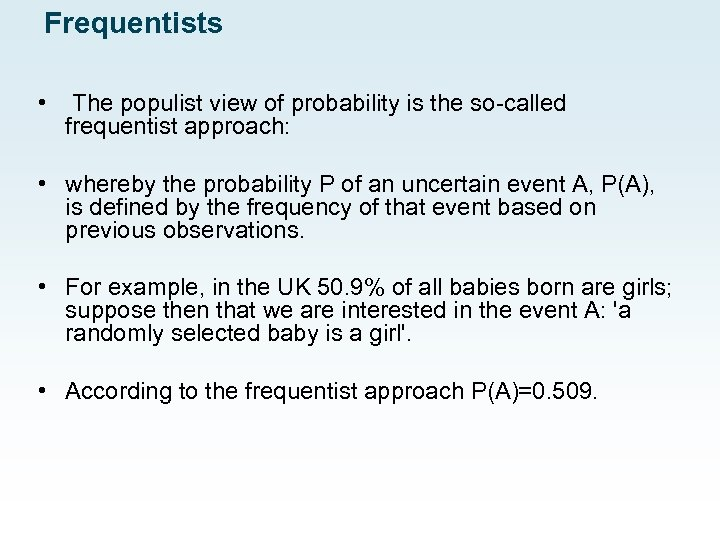Frequentists • The populist view of probability is the so-called frequentist approach: • whereby the probability P of an uncertain event A, P(A), is defined by the frequency of that event based on previous observations. • For example, in the UK 50. 9% of all babies born are girls; suppose then that we are interested in the event A: 'a randomly selected baby is a girl'. • According to the frequentist approach P(A)=0. 509.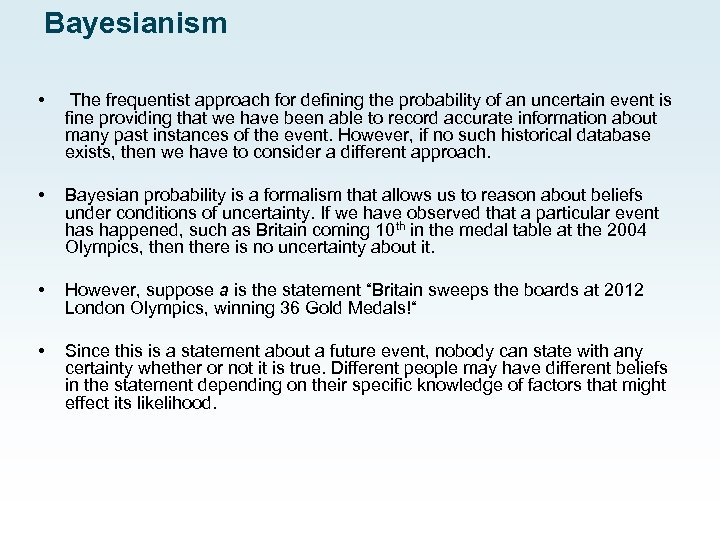Bayesianism • The frequentist approach for defining the probability of an uncertain event is fine providing that we have been able to record accurate information about many past instances of the event. However, if no such historical database exists, then we have to consider a different approach. • Bayesian probability is a formalism that allows us to reason about beliefs under conditions of uncertainty. If we have observed that a particular event has happened, such as Britain coming 10 th in the medal table at the 2004 Olympics, then there is no uncertainty about it. • However, suppose a is the statement “Britain sweeps the boards at 2012 London Olympics, winning 36 Gold Medals!“ • Since this is a statement about a future event, nobody can state with any certainty whether or not it is true. Different people may have different beliefs in the statement depending on their specific knowledge of factors that might effect its likelihood.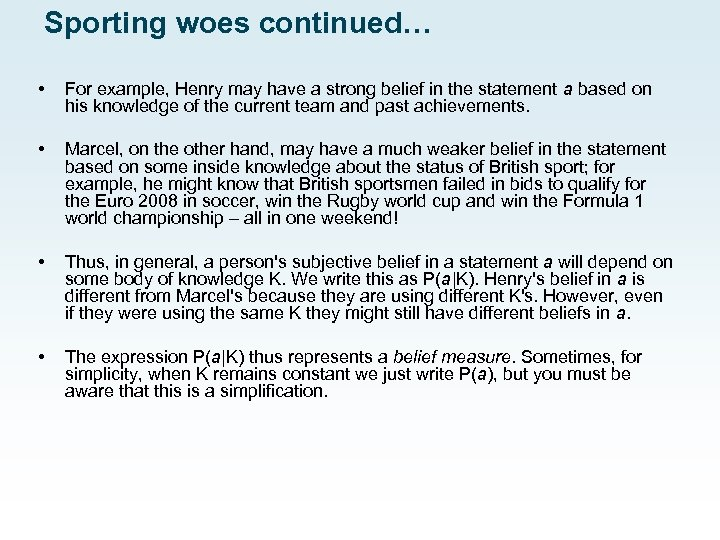Sporting woes continued… • For example, Henry may have a strong belief in the statement a based on his knowledge of the current team and past achievements. • Marcel, on the other hand, may have a much weaker belief in the statement based on some inside knowledge about the status of British sport; for example, he might know that British sportsmen failed in bids to qualify for the Euro 2008 in soccer, win the Rugby world cup and win the Formula 1 world championship – all in one weekend! • Thus, in general, a person's subjective belief in a statement a will depend on some body of knowledge K. We write this as P(a|K). Henry's belief in a is different from Marcel's because they are using different K's. However, even if they were using the same K they might still have different beliefs in a. • The expression P(a|K) thus represents a belief measure. Sometimes, for simplicity, when K remains constant we just write P(a), but you must be aware that this is a simplification.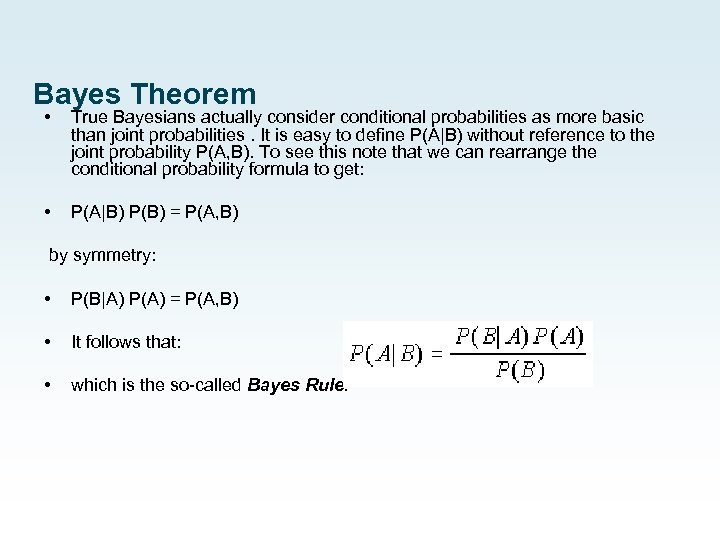Bayes Theorem • True Bayesians actually consider conditional probabilities as more basic than joint probabilities. It is easy to define P(A|B) without reference to the joint probability P(A, B). To see this note that we can rearrange the conditional probability formula to get: • P(A|B) P(B) = P(A, B) by symmetry: • P(B|A) P(A) = P(A, B) • It follows that: • which is the so-called Bayes Rule.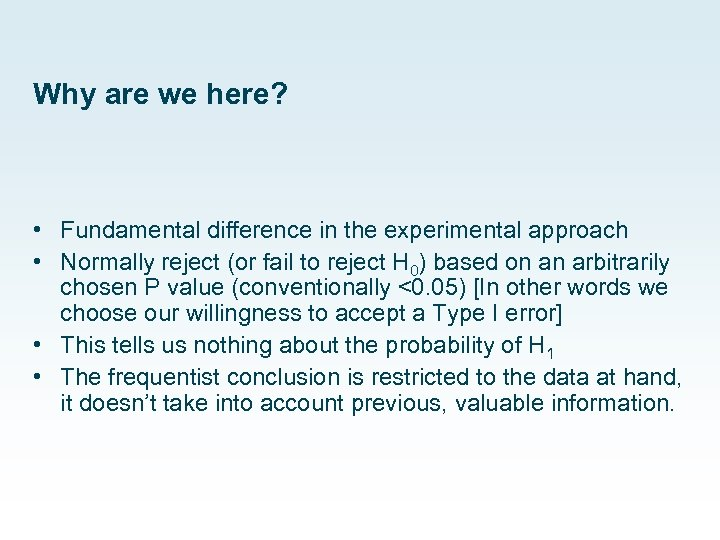Why are we here? • Fundamental difference in the experimental approach • Normally reject (or fail to reject H 0) based on an arbitrarily chosen P value (conventionally <0. 05) [In other words we choose our willingness to accept a Type I error] • This tells us nothing about the probability of H 1 • The frequentist conclusion is restricted to the data at hand, it doesn’t take into account previous, valuable information.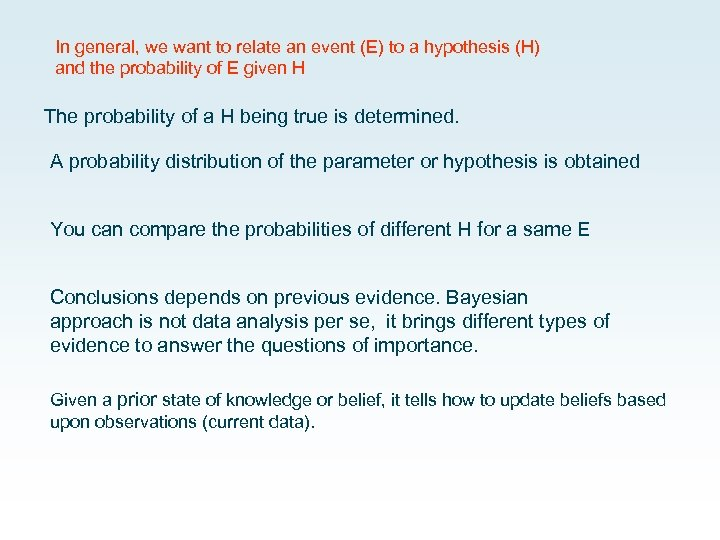In general, we want to relate an event (E) to a hypothesis (H) and the probability of E given H The probability of a H being true is determined. A probability distribution of the parameter or hypothesis is obtained You can compare the probabilities of different H for a same E Conclusions depends on previous evidence. Bayesian approach is not data analysis per se, it brings different types of evidence to answer the questions of importance. Given a prior state of knowledge or belief, it tells how to update beliefs based upon observations (current data).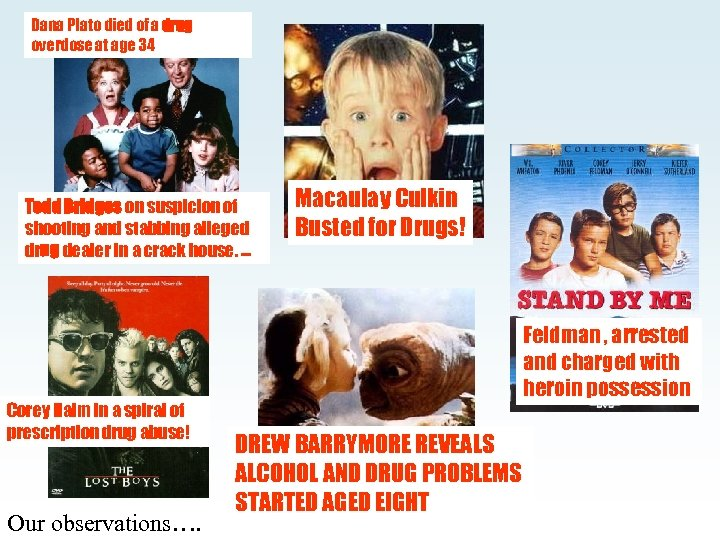Dana Plato died of a drug overdose at age 34 Todd Bridges on suspicion of shooting and stabbing alleged drug dealer in a crack house. . Corey Haim in a spiral of prescription drug abuse! Our observations…. Macaulay Culkin Busted for Drugs! Feldman , arrested and charged with heroin possession DREW BARRYMORE REVEALS ALCOHOL AND DRUG PROBLEMS STARTED AGED EIGHT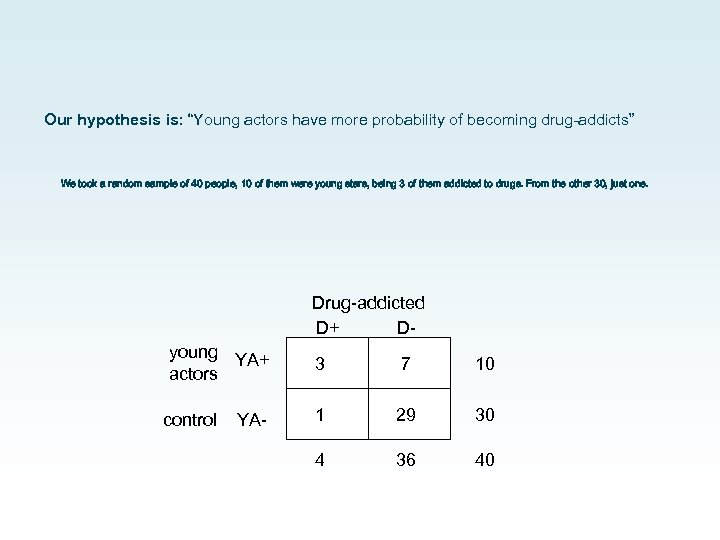Our hypothesis is: “Young actors have more probability of becoming drug-addicts” We took a random sample of 40 people, 10 of them were young stars, being 3 of them addicted to drugs. From the other 30, just one. Drug-addicted D+ Dyoung YA+ actors control YA- 3 7 10 1 29 30 4 36 40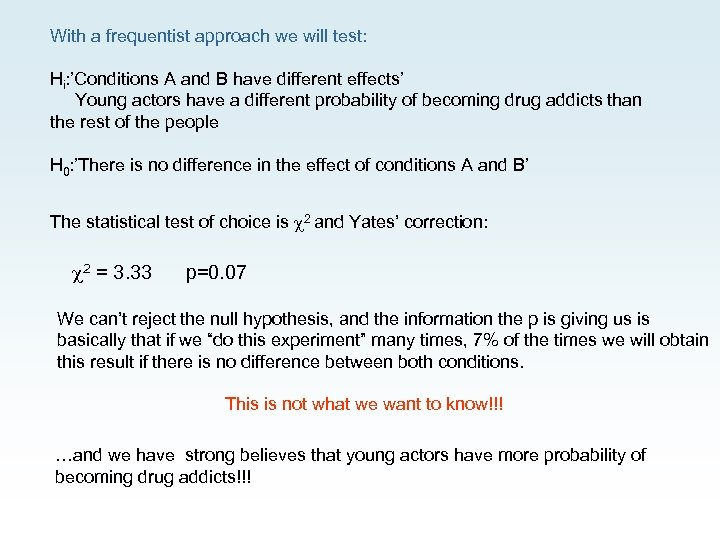With a frequentist approach we will test: Hi: ’Conditions A and B have different effects’ Young actors have a different probability of becoming drug addicts than the rest of the people H 0: ’There is no difference in the effect of conditions A and B’ The statistical test of choice is 2 and Yates’ correction: 2 = 3. 33 p=0. 07 We can’t reject the null hypothesis, and the information the p is giving us is basically that if we “do this experiment” many times, 7% of the times we will obtain this result if there is no difference between both conditions. This is not what we want to know!!! …and we have strong believes that young actors have more probability of becoming drug addicts!!!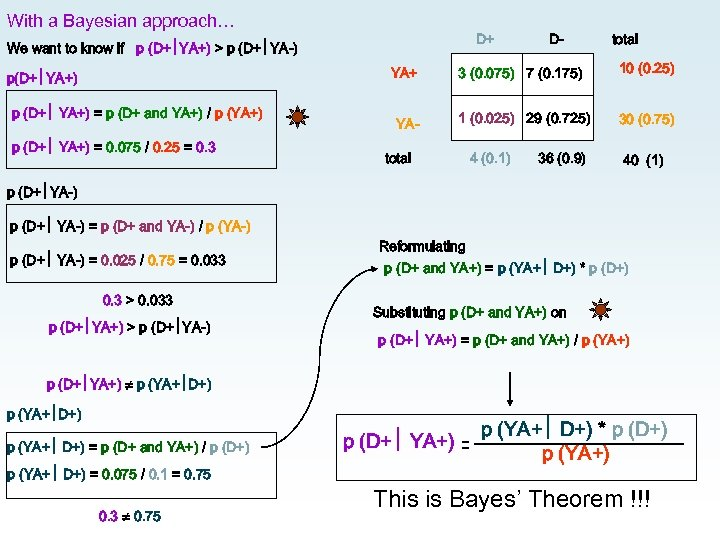With a Bayesian approach… D+ We want to know if p (D+ YA+) > p (D+ YA-) D- total YA+ p(D+ YA+) p (D+ YA+) = p (D+ and YA+) / p (YA+) p (D+ YA+) = 0. 075 / 0. 25 = 0. 3 3 (0. 075) 7 (0. 175) 10 (0. 25) YA- 1 (0. 025) 29 (0. 725) 30 (0. 75) total 4 (0. 1) 36 (0. 9) 40 (1) p (D+ YA-) = p (D+ and YA-) / p (YA-) p (D+ YA-) = 0. 025 / 0. 75 = 0. 033 0. 3 > 0. 033 p (D+ YA+) > p (D+ YA-) Reformulating p (D+ and YA+) = p (YA+ D+) * p (D+) Substituting p (D+ and YA+) on p (D+ YA+) = p (D+ and YA+) / p (YA+) p (D+ YA+) p (YA+ D+) = p (D+ and YA+) / p (D+) p (YA+ D+) = 0. 075 / 0. 1 = 0. 75 0. 3 0. 75 p (D+ YA+) p (YA+ D+) * p (D+) p (YA+) This is Bayes’ Theorem !!!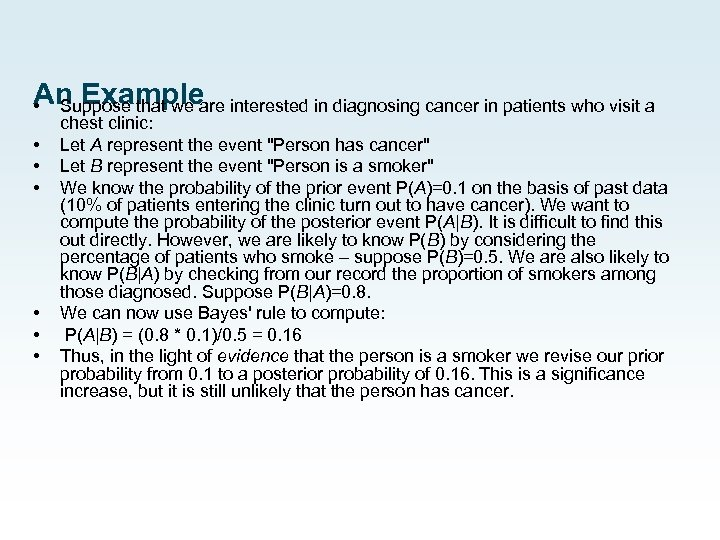An Example Suppose that we are interested in diagnosing cancer in patients who visit a • • chest clinic: Let A represent the event "Person has cancer" Let B represent the event "Person is a smoker" We know the probability of the prior event P(A)=0. 1 on the basis of past data (10% of patients entering the clinic turn out to have cancer). We want to compute the probability of the posterior event P(A|B). It is difficult to find this out directly. However, we are likely to know P(B) by considering the percentage of patients who smoke – suppose P(B)=0. 5. We are also likely to know P(B|A) by checking from our record the proportion of smokers among those diagnosed. Suppose P(B|A)=0. 8. We can now use Bayes' rule to compute: P(A|B) = (0. 8 * 0. 1)/0. 5 = 0. 16 Thus, in the light of evidence that the person is a smoker we revise our prior probability from 0. 1 to a posterior probability of 0. 16. This is a significance increase, but it is still unlikely that the person has cancer.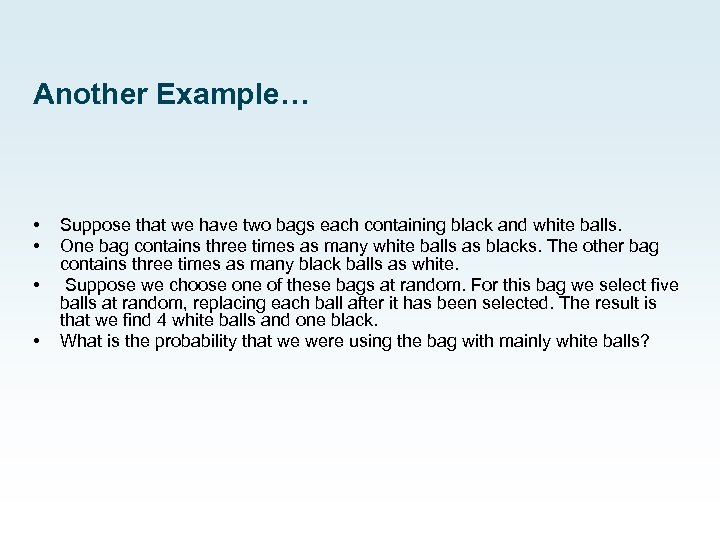Another Example… • • Suppose that we have two bags each containing black and white balls. One bag contains three times as many white balls as blacks. The other bag contains three times as many black balls as white. Suppose we choose one of these bags at random. For this bag we select five balls at random, replacing each ball after it has been selected. The result is that we find 4 white balls and one black. What is the probability that we were using the bag with mainly white balls?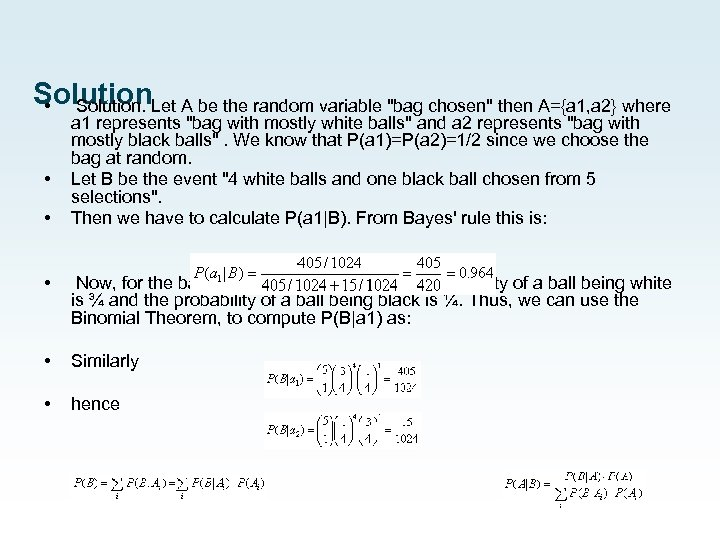Solution • Solution. Let A be the random variable "bag chosen" then A={a 1, a 2} where • • a 1 represents "bag with mostly white balls" and a 2 represents "bag with mostly black balls". We know that P(a 1)=P(a 2)=1/2 since we choose the bag at random. Let B be the event "4 white balls and one black ball chosen from 5 selections". Then we have to calculate P(a 1|B). From Bayes' rule this is: • Now, for the bag with mostly white balls the probability of a ball being white is ¾ and the probability of a ball being black is ¼. Thus, we can use the Binomial Theorem, to compute P(B|a 1) as: • Similarly • hence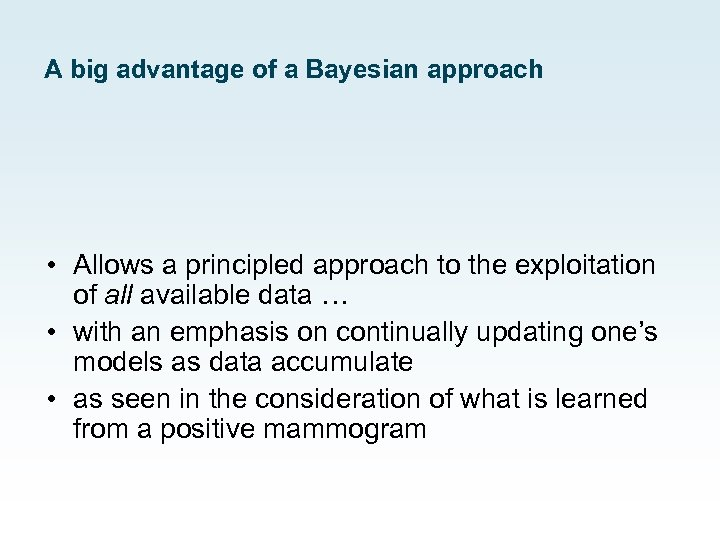A big advantage of a Bayesian approach • Allows a principled approach to the exploitation of all available data … • with an emphasis on continually updating one’s models as data accumulate • as seen in the consideration of what is learned from a positive mammogram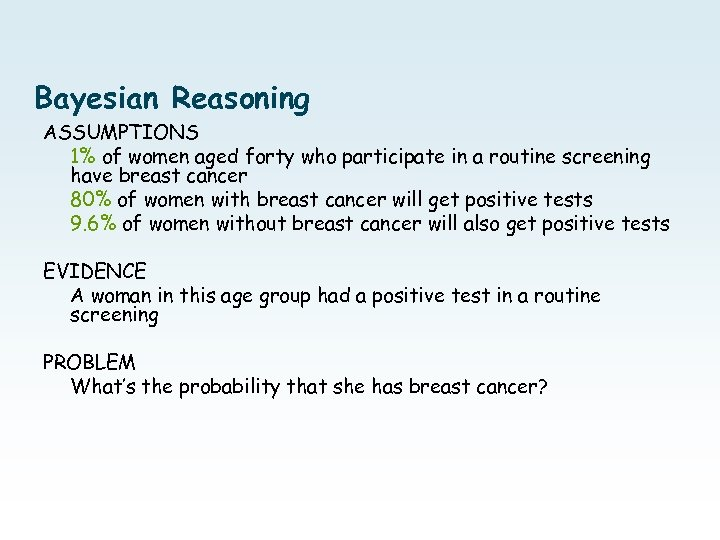Bayesian Reasoning ASSUMPTIONS 1% of women aged forty who participate in a routine screening have breast cancer 80% of women with breast cancer will get positive tests 9. 6% of women without breast cancer will also get positive tests EVIDENCE A woman in this age group had a positive test in a routine screening PROBLEM What’s the probability that she has breast cancer?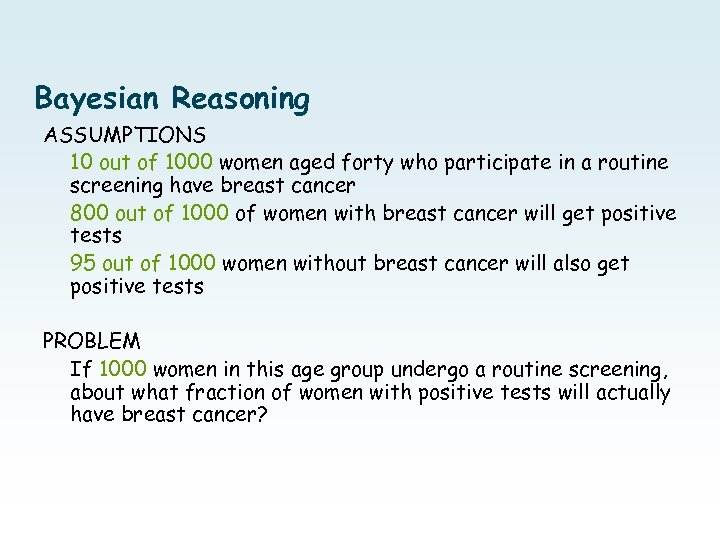Bayesian Reasoning ASSUMPTIONS 10 out of 1000 women aged forty who participate in a routine screening have breast cancer 800 out of 1000 of women with breast cancer will get positive tests 95 out of 1000 women without breast cancer will also get positive tests PROBLEM If 1000 women in this age group undergo a routine screening, about what fraction of women with positive tests will actually have breast cancer?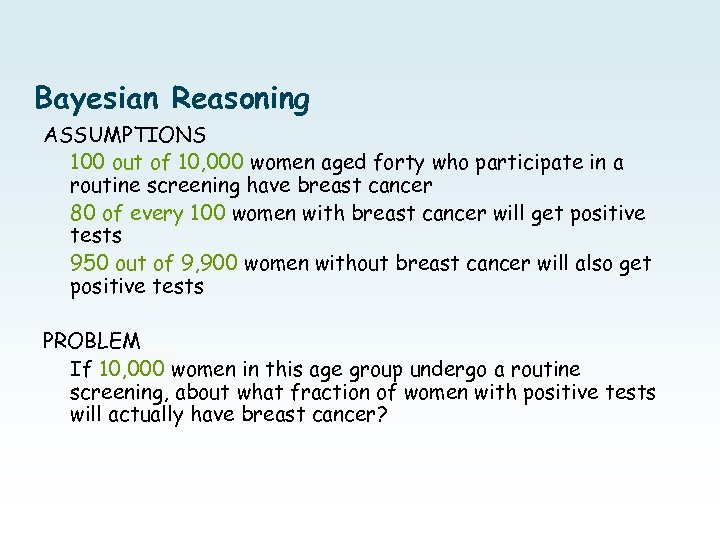Bayesian Reasoning ASSUMPTIONS 100 out of 10, 000 women aged forty who participate in a routine screening have breast cancer 80 of every 100 women with breast cancer will get positive tests 950 out of 9, 900 women without breast cancer will also get positive tests PROBLEM If 10, 000 women in this age group undergo a routine screening, about what fraction of women with positive tests will actually have breast cancer?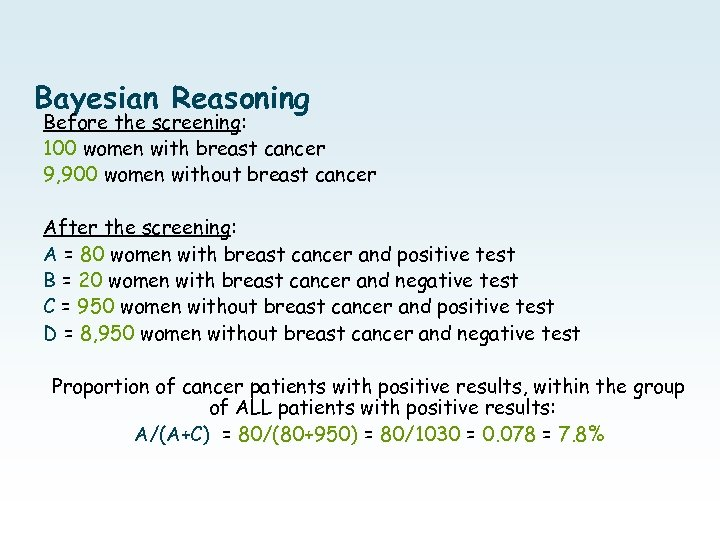Bayesian Reasoning Before the screening: 100 women with breast cancer 9, 900 women without breast cancer After the screening: A = 80 women with breast cancer and positive test B = 20 women with breast cancer and negative test C = 950 women without breast cancer and positive test D = 8, 950 women without breast cancer and negative test Proportion of cancer patients with positive results, within the group of ALL patients with positive results: A/(A+C) = 80/(80+950) = 80/1030 = 0. 078 = 7. 8%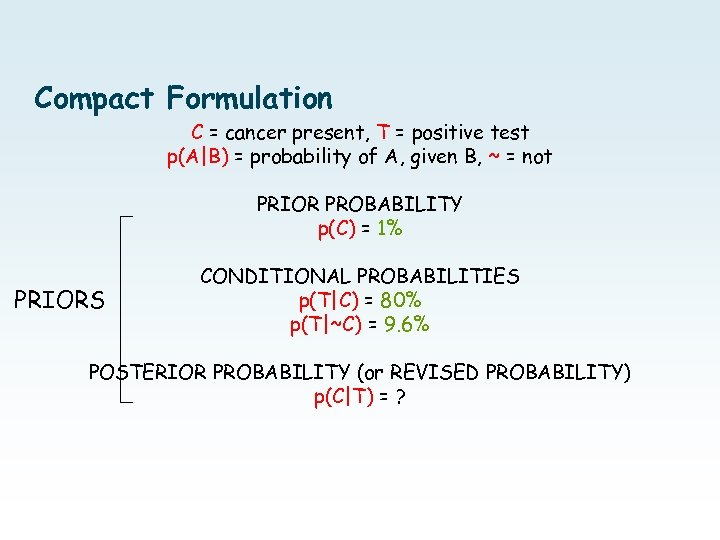Compact Formulation C = cancer present, T = positive test p(A|B) = probability of A, given B, ~ = not PRIOR PROBABILITY p(C) = 1% PRIORS CONDITIONAL PROBABILITIES p(T|C) = 80% p(T|~C) = 9. 6% POSTERIOR PROBABILITY (or REVISED PROBABILITY) p(C|T) = ?Bayesian Reasoning Before the screening: 100 women with breast cancer 9, 900 women without breast cancer After the screening: A = 80 women with breast cancer and positive test B = 20 women with breast cancer and negative test C = 950 women without breast cancer and positive test D = 8, 950 women without breast cancer and negative test Proportion of cancer patients with positive results, within the group of ALL patients with positive results: A/(A+C) = 80/(80+950) = 80/1030 = 0. 078 = 7. 8%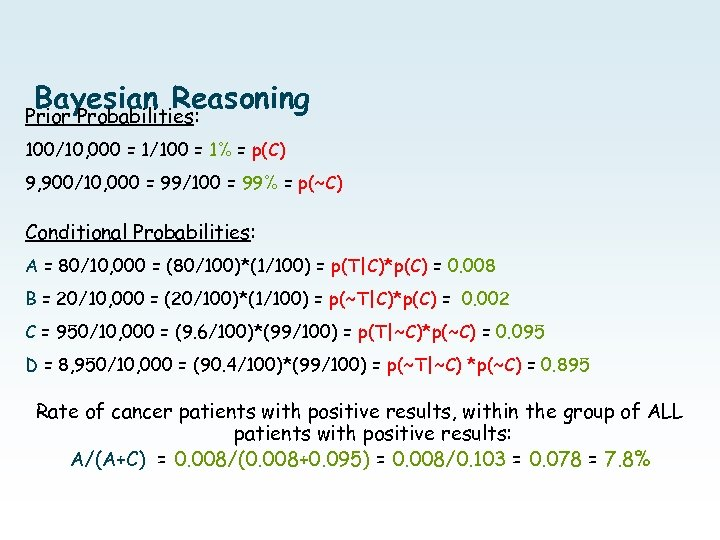Bayesian Reasoning Prior Probabilities: 100/10, 000 = 1/100 = 1% = p(C) 9, 900/10, 000 = 99/100 = 99% = p(~C) Conditional Probabilities: A = 80/10, 000 = (80/100)*(1/100) = p(T|C)*p(C) = 0. 008 B = 20/10, 000 = (20/100)*(1/100) = p(~T|C)*p(C) = 0. 002 C = 950/10, 000 = (9. 6/100)*(99/100) = p(T|~C)*p(~C) = 0. 095 D = 8, 950/10, 000 = (90. 4/100)*(99/100) = p(~T|~C) *p(~C) = 0. 895 Rate of cancer patients with positive results, within the group of ALL patients with positive results: A/(A+C) = 0. 008/(0. 008+0. 095) = 0. 008/0. 103 = 0. 078 = 7. 8%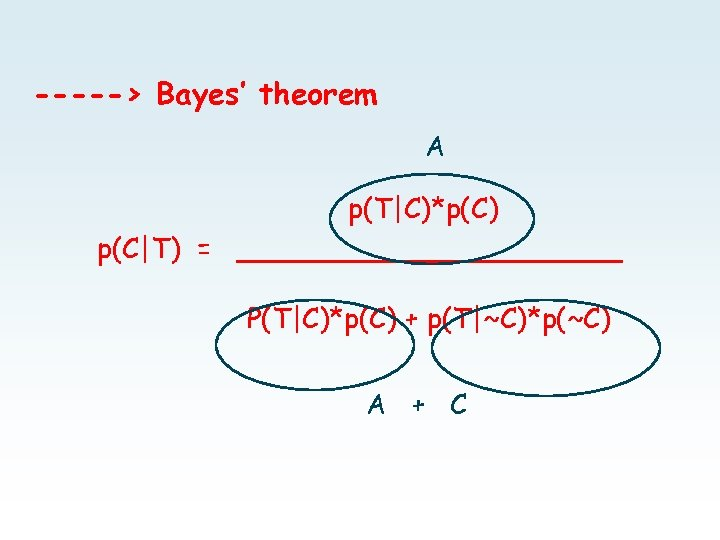-----> Bayes’ theorem A p(T|C)*p(C) p(C|T) = ___________ P(T|C)*p(C) + p(T|~C)*p(~C) A + C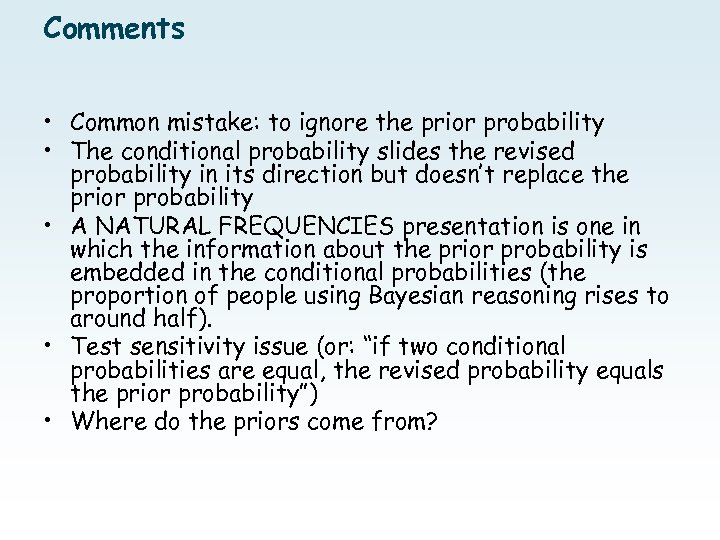Comments • Common mistake: to ignore the prior probability • The conditional probability slides the revised probability in its direction but doesn’t replace the prior probability • A NATURAL FREQUENCIES presentation is one in which the information about the prior probability is embedded in the conditional probabilities (the proportion of people using Bayesian reasoning rises to around half). • Test sensitivity issue (or: “if two conditional probabilities are equal, the revised probability equals the prior probability”) • Where do the priors come from?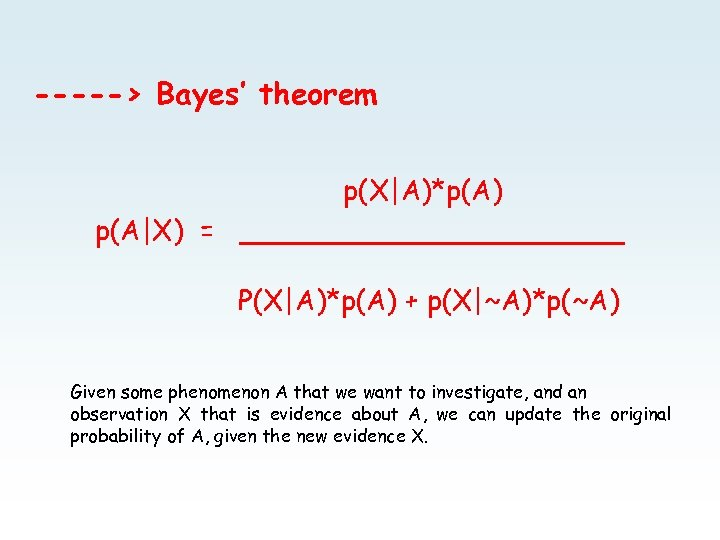-----> Bayes’ theorem p(X|A)*p(A) p(A|X) = ___________ P(X|A)*p(A) + p(X|~A)*p(~A) Given some phenomenon A that we want to investigate, and an observation X that is evidence about A, we can update the original probability of A, given the new evidence X.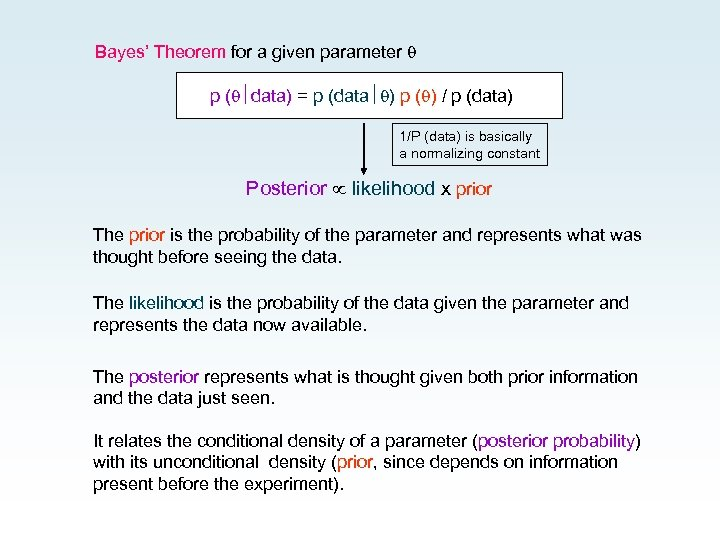Bayes’ Theorem for a given parameter p ( data) = p (data ) p ( ) / p (data) 1/P (data) is basically a normalizing constant Posterior likelihood x prior The prior is the probability of the parameter and represents what was thought before seeing the data. The likelihood is the probability of the data given the parameter and represents the data now available. The posterior represents what is thought given both prior information and the data just seen. It relates the conditional density of a parameter (posterior probability) with its unconditional density (prior, since depends on information present before the experiment).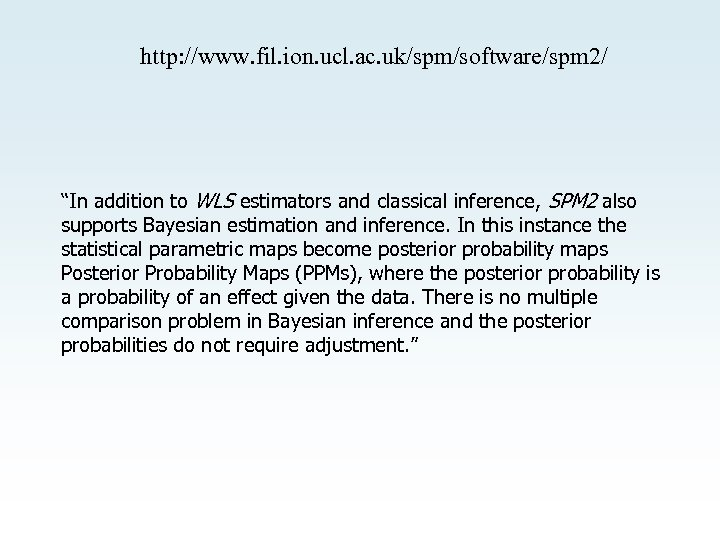http: //www. fil. ion. ucl. ac. uk/spm/software/spm 2/ “In addition to WLS estimators and classical inference, SPM 2 also supports Bayesian estimation and inference. In this instance the statistical parametric maps become posterior probability maps Posterior Probability Maps (PPMs), where the posterior probability is a probability of an effect given the data. There is no multiple comparison problem in Bayesian inference and the posterior probabilities do not require adjustment. ”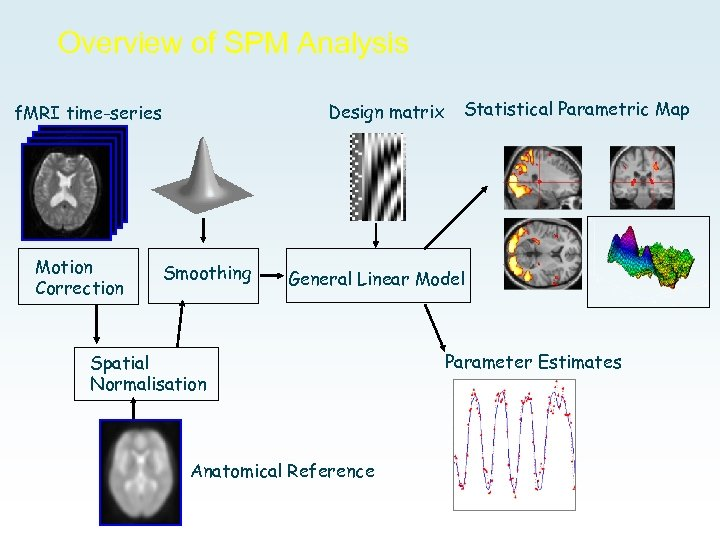Overview of SPM Analysis Design matrix f. MRI time-series Motion Correction Smoothing Statistical Parametric Map General Linear Model Spatial Normalisation Anatomical Reference Parameter Estimates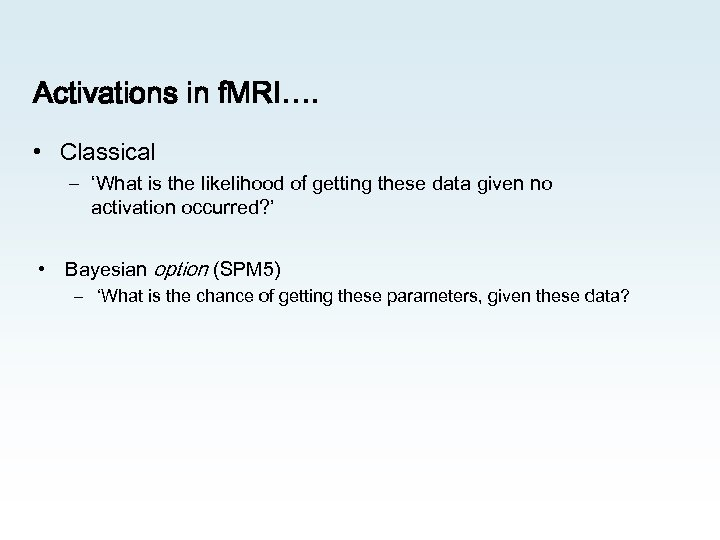Activations in f. MRI…. • Classical – ‘What is the likelihood of getting these data given no activation occurred? ’ • Bayesian option (SPM 5) – ‘What is the chance of getting these parameters, given these data?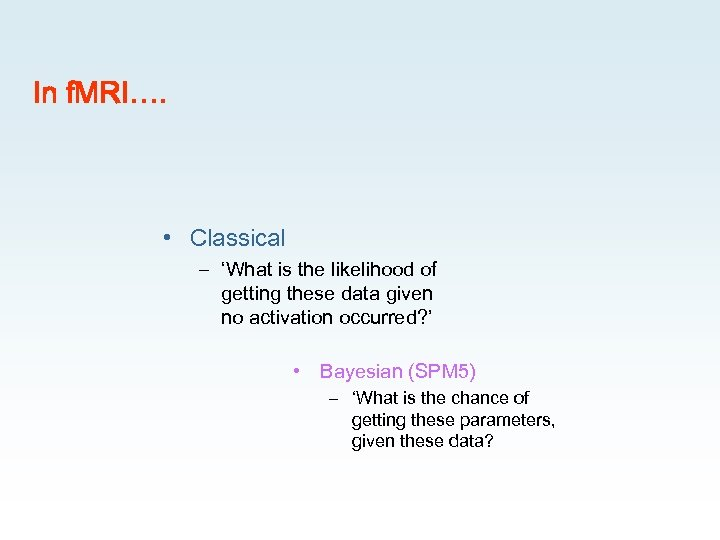In f. MRI…. • Classical – ‘What is the likelihood of getting these data given no activation occurred? ’ • Bayesian (SPM 5) – ‘What is the chance of getting these parameters, given these data?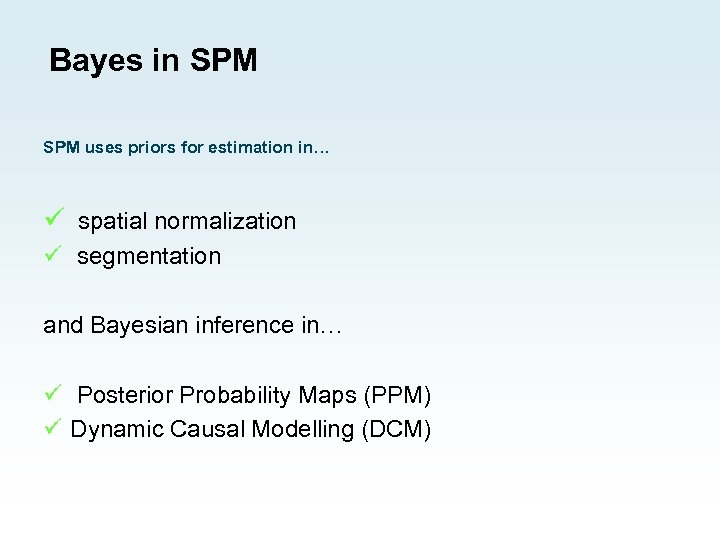Bayes in SPM uses priors for estimation in… ü spatial normalization ü segmentation and Bayesian inference in… ü Posterior Probability Maps (PPM) ü Dynamic Causal Modelling (DCM)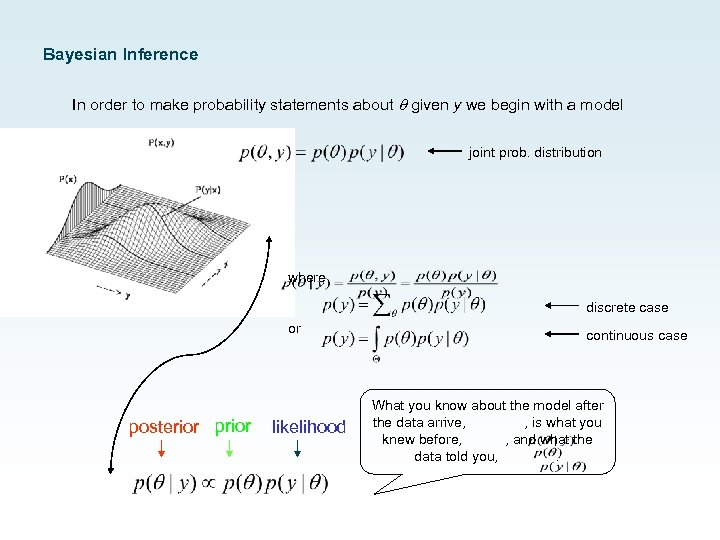Bayesian Inference In order to make probability statements about given y we begin with a model joint prob. distribution where discrete case or posterior prior likelihood continuous case What you know about the model after the data arrive, , is what you knew before, , and what the data told you, .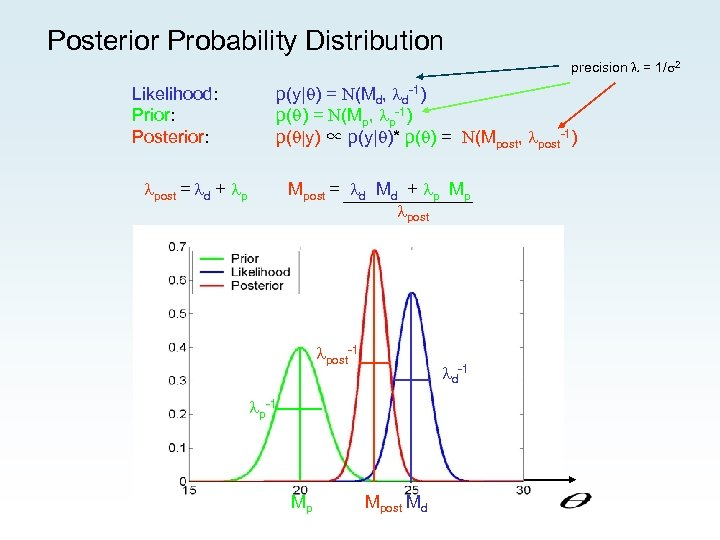Posterior Probability Distribution precision = 1/ 2 Likelihood: Prior: Posterior: p(y| ) = N(Md, d-1) p( ) = N(Mp, p-1) p( |y) ∝ p(y| )* p( ) = N(Mpost, post-1) post = d + p Mpost = d Md + p Mp post-1 d-1 p-1 Mp Mpost Md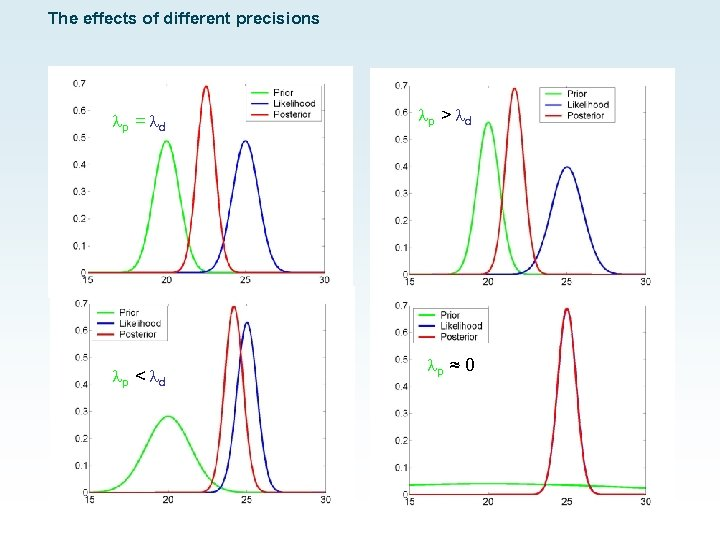The effects of different precisions p = d p < d p > d p ≈ 0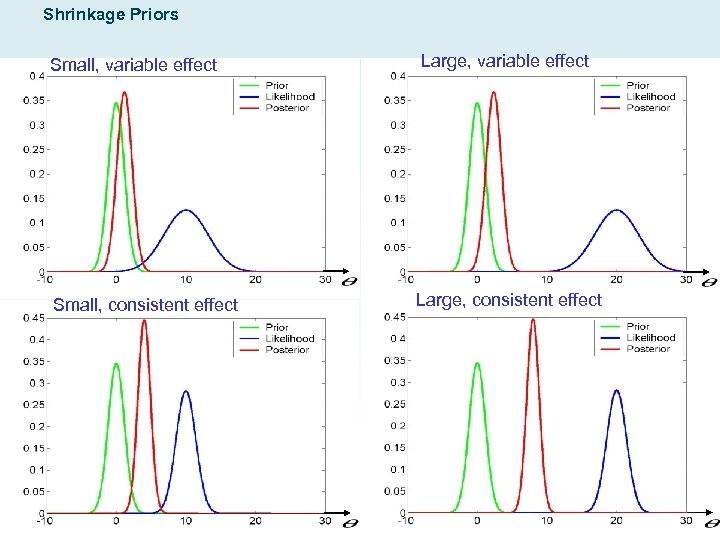Shrinkage Priors Small, variable effect Large, variable effect Small, consistent effect Large, consistent effect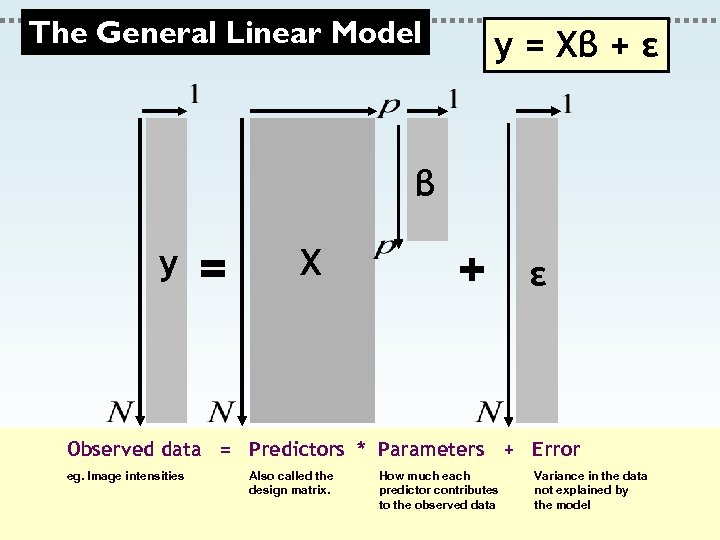The General Linear Model y = Xβ + ε β y = X + ε Observed data = Predictors * Parameters + Error eg. Image intensities Also called the design matrix. How much each predictor contributes to the observed data Variance in the data not explained by the model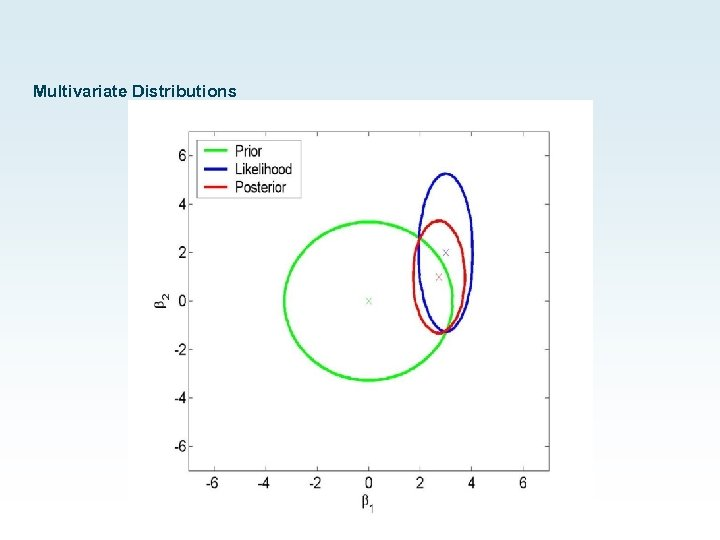Multivariate Distributions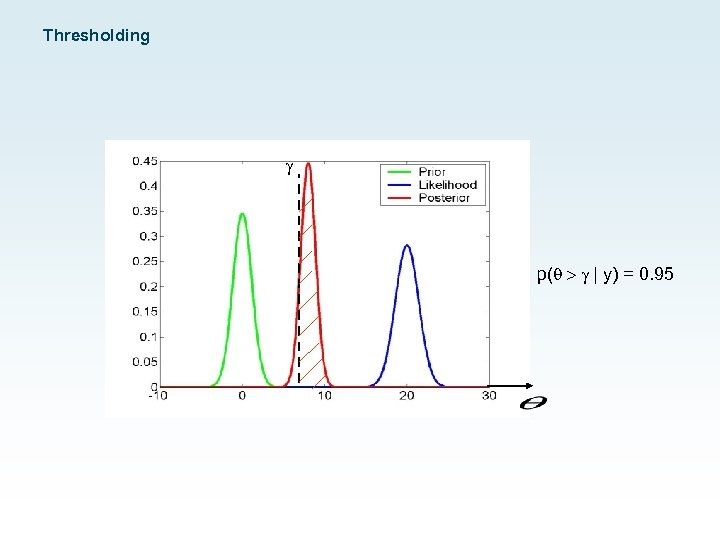Thresholding g p( > g | y) = 0. 95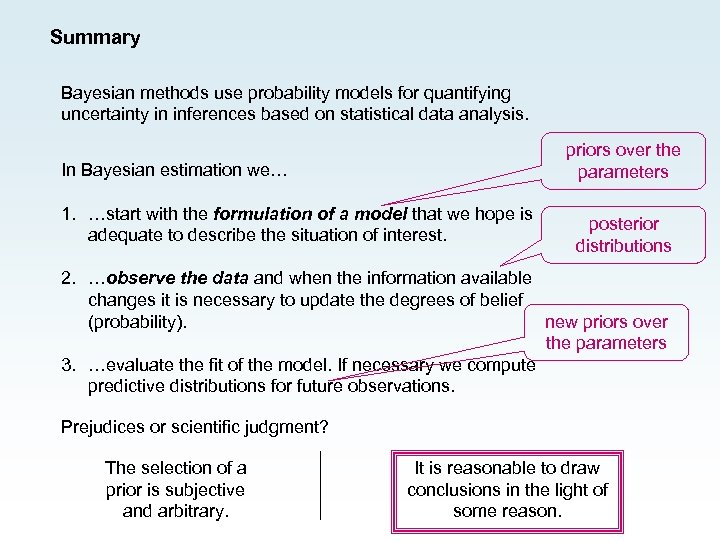Summary Bayesian methods use probability models for quantifying uncertainty in inferences based on statistical data analysis. priors over the parameters In Bayesian estimation we… 1. …start with the formulation of a model that we hope is adequate to describe the situation of interest. posterior distributions 2. …observe the data and when the information available changes it is necessary to update the degrees of belief (probability). new priors over the parameters 3. …evaluate the fit of the model. If necessary we compute predictive distributions for future observations. Prejudices or scientific judgment? The selection of a prior is subjective and arbitrary. It is reasonable to draw conclusions in the light of some reason.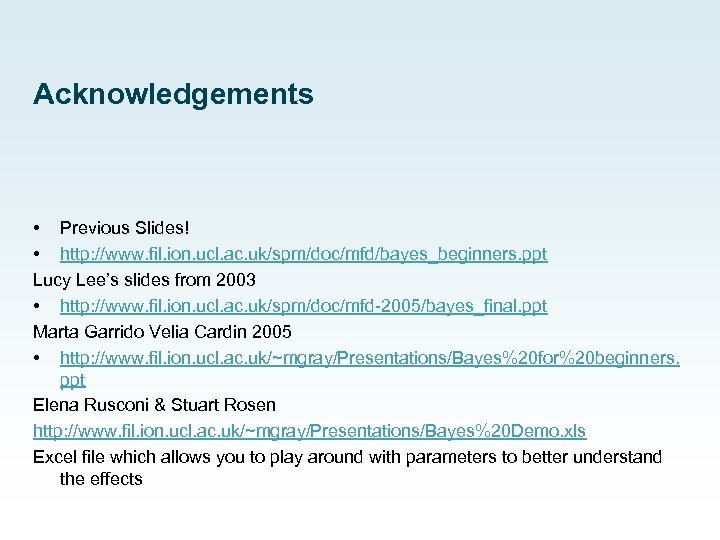Acknowledgements • Previous Slides! • http: //www. fil. ion. ucl. ac. uk/spm/doc/mfd/bayes_beginners. ppt Lucy Lee’s slides from 2003 • http: //www. fil. ion. ucl. ac. uk/spm/doc/mfd-2005/bayes_final. ppt Marta Garrido Velia Cardin 2005 • http: //www. fil. ion. ucl. ac. uk/~mgray/Presentations/Bayes%20 for%20 beginners. ppt Elena Rusconi & Stuart Rosen http: //www. fil. ion. ucl. ac. uk/~mgray/Presentations/Bayes%20 Demo. xls Excel file which allows you to play around with parameters to better understand the effects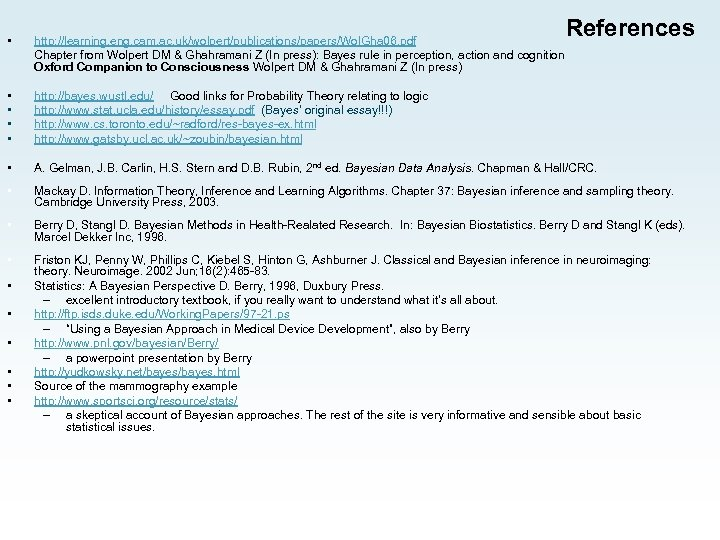References • http: //learning. eng. cam. ac. uk/wolpert/publications/papers/Wol. Gha 06. pdf Chapter from Wolpert DM & Ghahramani Z (In press): Bayes rule in perception, action and cognition Oxford Companion to Consciousness Wolpert DM & Ghahramani Z (In press) • • http: //bayes. wustl. edu/ Good links for Probability Theory relating to logic http: //www. stat. ucla. edu/history/essay. pdf (Bayes’ original essay!!!) http: //www. cs. toronto. edu/~radford/res-bayes-ex. html http: //www. gatsby. ucl. ac. uk/~zoubin/bayesian. html • A. Gelman, J. B. Carlin, H. S. Stern and D. B. Rubin, 2 nd ed. Bayesian Data Analysis. Chapman & Hall/CRC. • Mackay D. Information Theory, Inference and Learning Algorithms. Chapter 37: Bayesian inference and sampling theory. Cambridge University Press, 2003. • Berry D, Stangl D. Bayesian Methods in Health-Realated Research. In: Bayesian Biostatistics. Berry D and Stangl K (eds). Marcel Dekker Inc, 1996. • Friston KJ, Penny W, Phillips C, Kiebel S, Hinton G, Ashburner J. Classical and Bayesian inference in neuroimaging: theory. Neuroimage. 2002 Jun; 16(2): 465 -83. Statistics: A Bayesian Perspective D. Berry, 1996, Duxbury Press. – excellent introductory textbook, if you really want to understand what it’s all about. http: //ftp. isds. duke. edu/Working. Papers/97 -21. ps – “Using a Bayesian Approach in Medical Device Development”, also by Berry http: //www. pnl. gov/bayesian/Berry/ – a powerpoint presentation by Berry http: //yudkowsky. net/bayes. html Source of the mammography example http: //www. sportsci. org/resource/stats/ – a skeptical account of Bayesian approaches. The rest of the site is very informative and sensible about basic statistical issues. • • •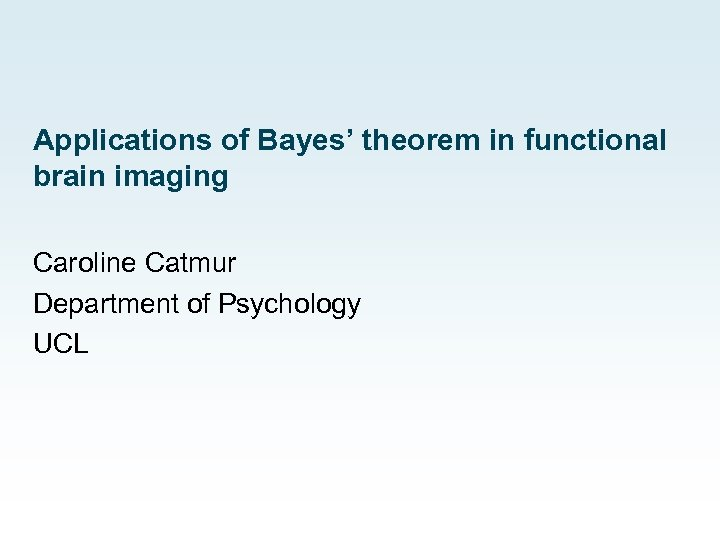Applications of Bayes’ theorem in functional brain imaging Caroline Catmur Department of Psychology UCL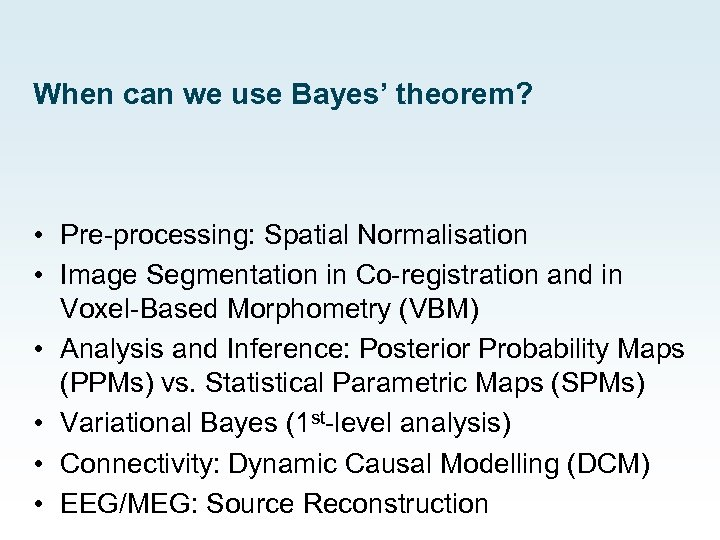When can we use Bayes’ theorem? • Pre-processing: Spatial Normalisation • Image Segmentation in Co-registration and in Voxel-Based Morphometry (VBM) • Analysis and Inference: Posterior Probability Maps (PPMs) vs. Statistical Parametric Maps (SPMs) • Variational Bayes (1 st-level analysis) • Connectivity: Dynamic Causal Modelling (DCM) • EEG/MEG: Source Reconstruction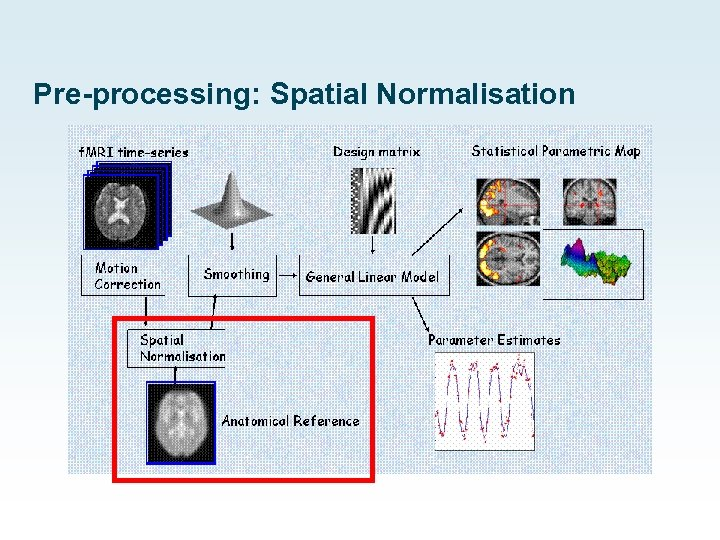Pre-processing: Spatial Normalisation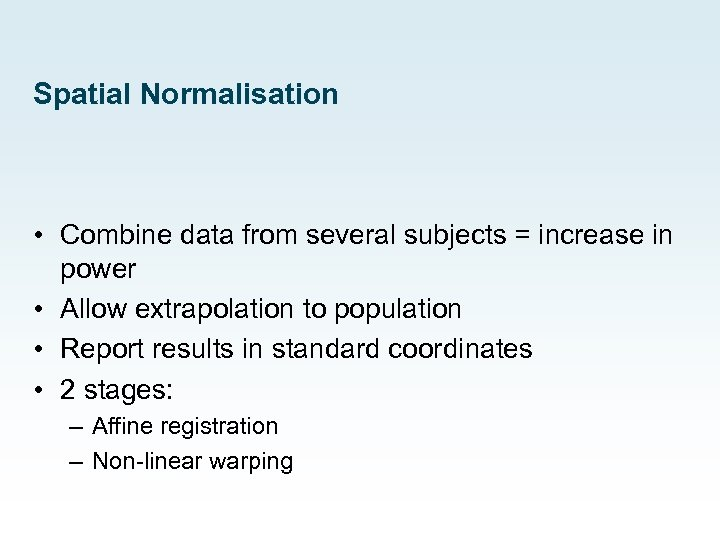Spatial Normalisation • Combine data from several subjects = increase in power • Allow extrapolation to population • Report results in standard coordinates • 2 stages: – Affine registration – Non-linear warping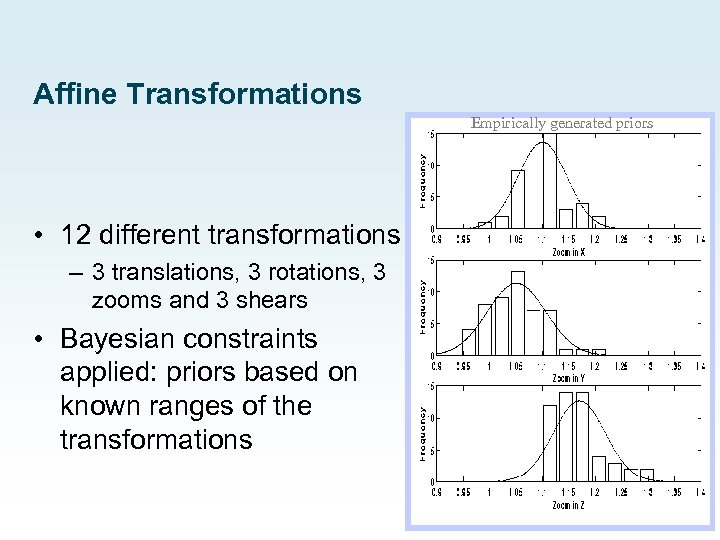Affine Transformations Empirically generated priors • 12 different transformations – 3 translations, 3 rotations, 3 zooms and 3 shears • Bayesian constraints applied: priors based on known ranges of the transformations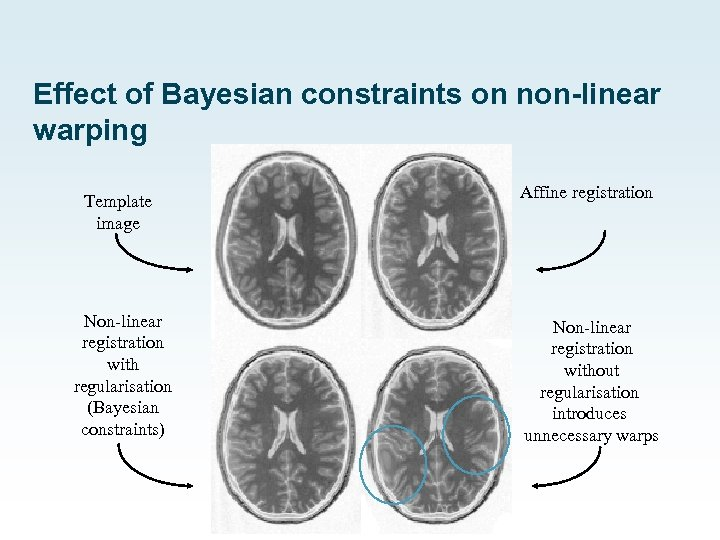Effect of Bayesian constraints on non-linear warping Template image Affine registration Non-linear registration with regularisation (Bayesian constraints) Non-linear registration without regularisation introduces unnecessary warps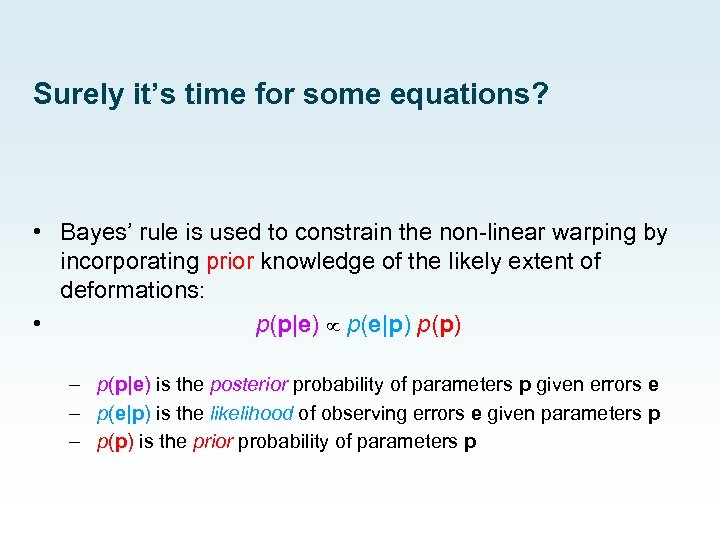Surely it’s time for some equations? • Bayes’ rule is used to constrain the non-linear warping by incorporating prior knowledge of the likely extent of deformations: • p(p|e) p(e|p) p(p) – p(p|e) is the posterior probability of parameters p given errors e – p(e|p) is the likelihood of observing errors e given parameters p – p(p) is the prior probability of parameters p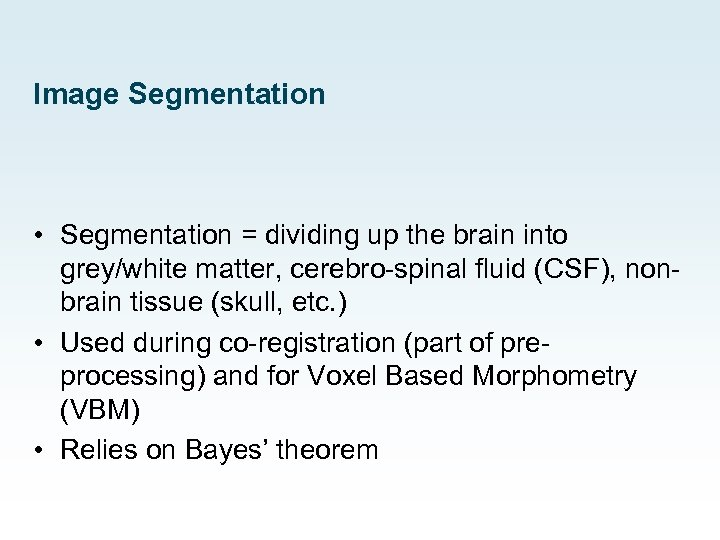Image Segmentation • Segmentation = dividing up the brain into grey/white matter, cerebro-spinal fluid (CSF), nonbrain tissue (skull, etc. ) • Used during co-registration (part of preprocessing) and for Voxel Based Morphometry (VBM) • Relies on Bayes’ theorem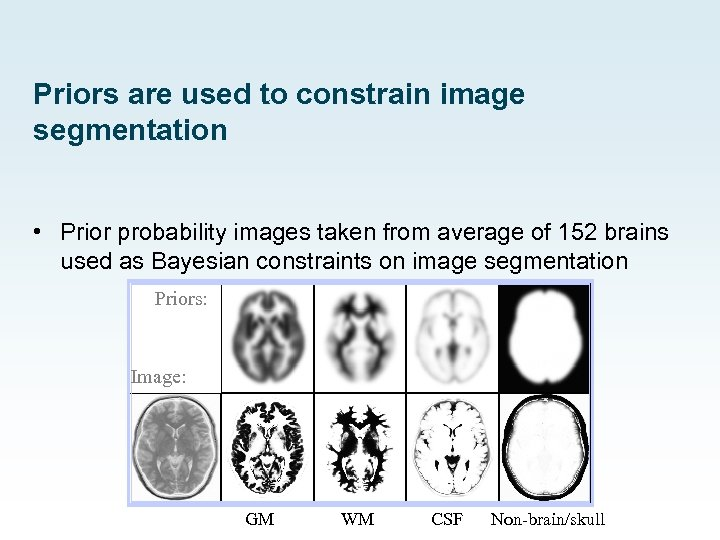Priors are used to constrain image segmentation • Prior probability images taken from average of 152 brains used as Bayesian constraints on image segmentation Priors: Image: GM WM CSF Non-brain/skull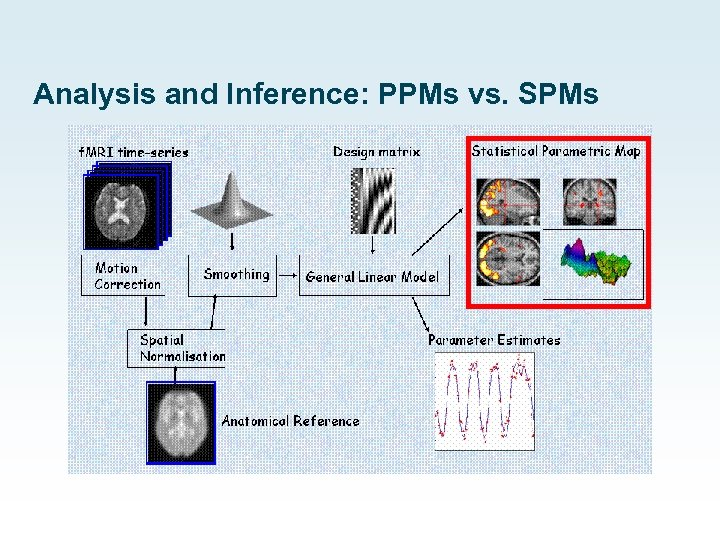Analysis and Inference: PPMs vs. SPMs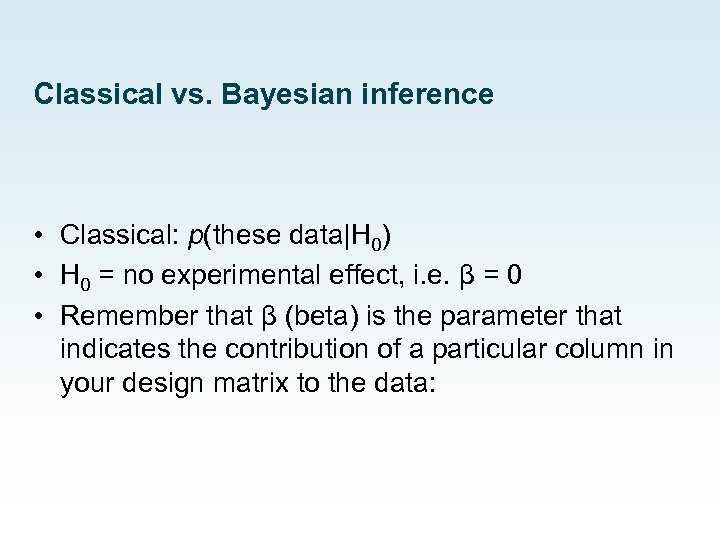Classical vs. Bayesian inference • Classical: p(these data|H 0) • H 0 = no experimental effect, i. e. β = 0 • Remember that β (beta) is the parameter that indicates the contribution of a particular column in your design matrix to the data: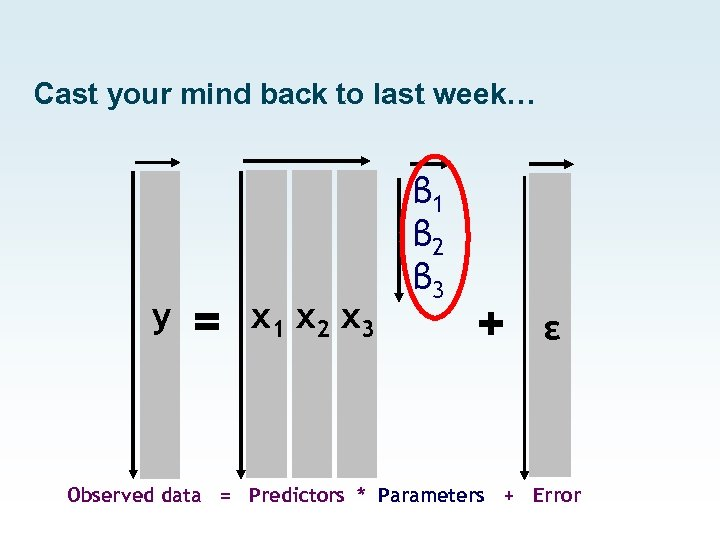Cast your mind back to last week… y = x 1 x 2 x 3 β 1 β 2 β 3 + ε Observed data = Predictors * Parameters + Error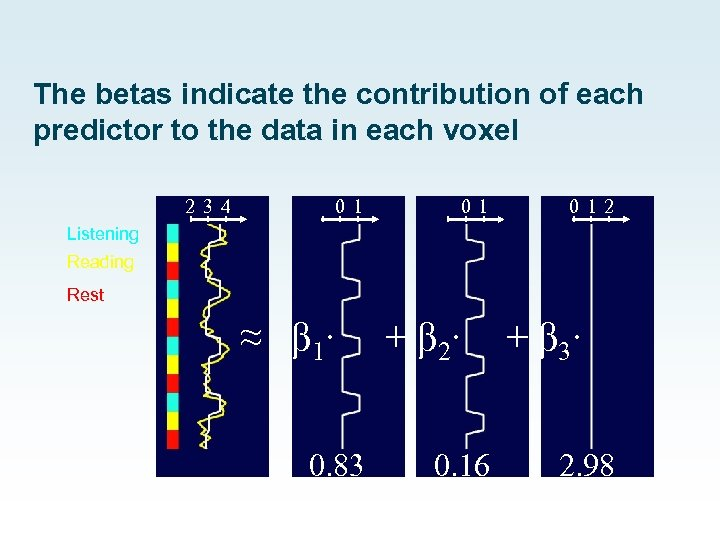The betas indicate the contribution of each predictor to the data in each voxel 2 34 01 01 0 12 Listening Reading Rest ≈ β 1∙ 0. 83 + β 2∙ 0. 16 + β 3∙ 2. 98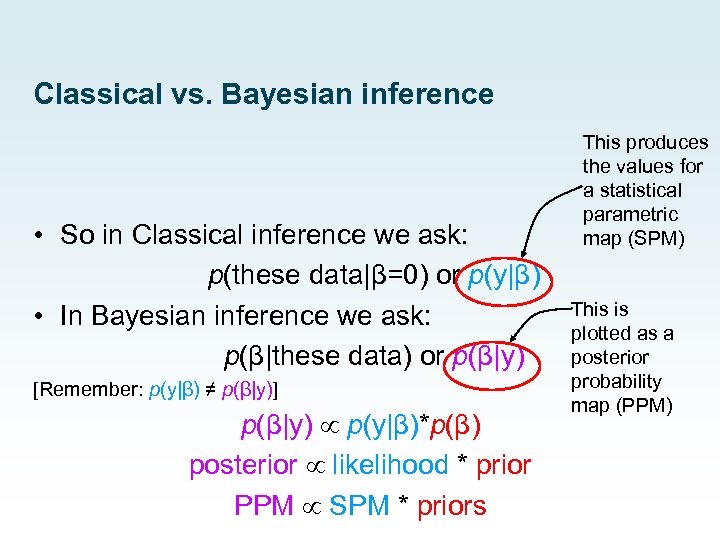Classical vs. Bayesian inference • So in Classical inference we ask: p(these data|β=0) or p(y|β) • In Bayesian inference we ask: p(β|these data) or p(β|y) [Remember: p(y|β) ≠ p(β|y)] p(β|y) p(y|β)*p(β) posterior likelihood * prior PPM SPM * priors This produces the values for a statistical parametric map (SPM) This is plotted as a posterior probability map (PPM)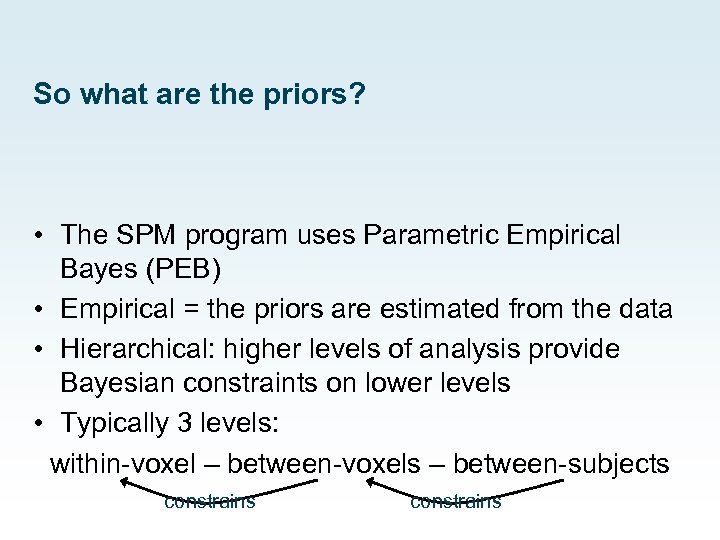So what are the priors? • The SPM program uses Parametric Empirical Bayes (PEB) • Empirical = the priors are estimated from the data • Hierarchical: higher levels of analysis provide Bayesian constraints on lower levels • Typically 3 levels: within-voxel – between-voxels – between-subjects constrains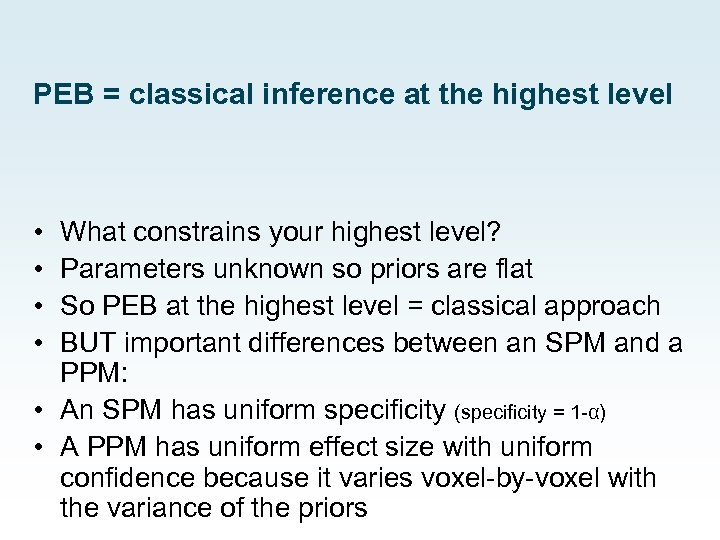PEB = classical inference at the highest level • • What constrains your highest level? Parameters unknown so priors are flat So PEB at the highest level = classical approach BUT important differences between an SPM and a PPM: • An SPM has uniform specificity (specificity = 1 -α) • A PPM has uniform effect size with uniform confidence because it varies voxel-by-voxel with the variance of the priorsPutting it another way: PPMs vs. SPMs • When you threshold a PPM you are specifying a desired effect size • But for an SPM, if a voxel survives thresholding it could be a big effect with relatively high variance but it could also be a small effect with low variance… • … and as you increase the number of scans and/or subjects, probability of a very small effect surviving increases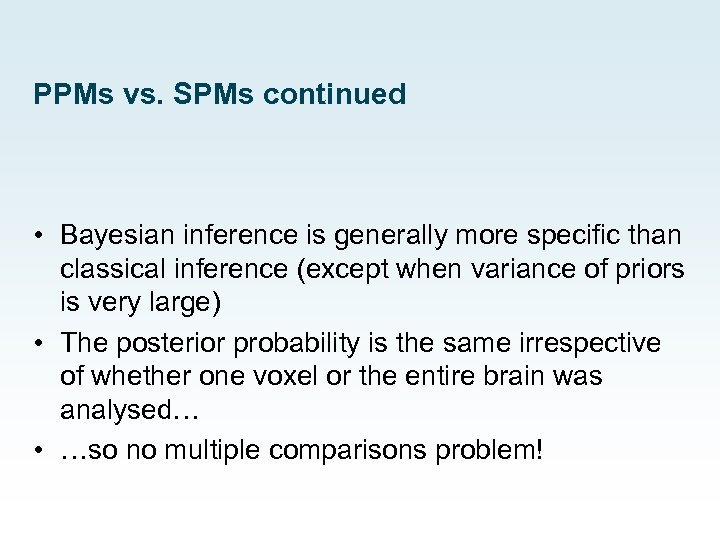PPMs vs. SPMs continued • Bayesian inference is generally more specific than classical inference (except when variance of priors is very large) • The posterior probability is the same irrespective of whether one voxel or the entire brain was analysed… • …so no multiple comparisons problem!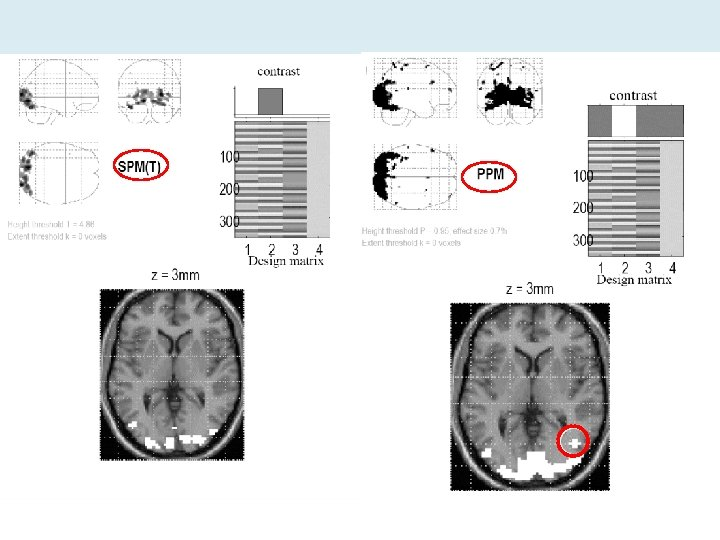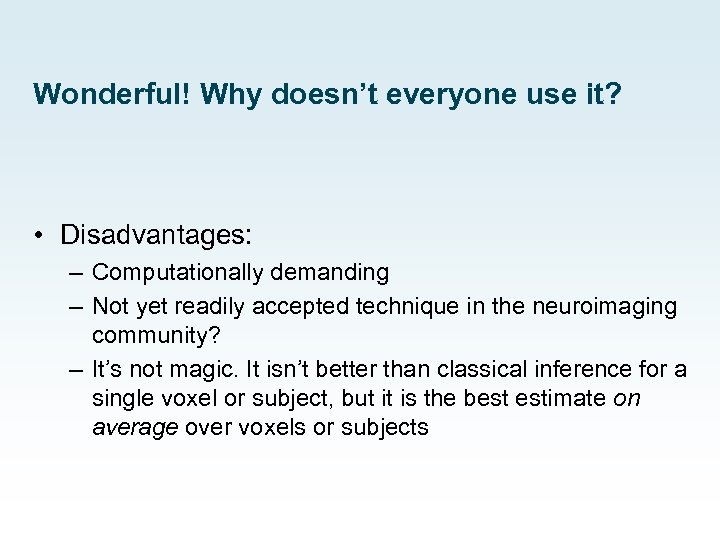Wonderful! Why doesn’t everyone use it? • Disadvantages: – Computationally demanding – Not yet readily accepted technique in the neuroimaging community? – It’s not magic. It isn’t better than classical inference for a single voxel or subject, but it is the best estimate on average over voxels or subjects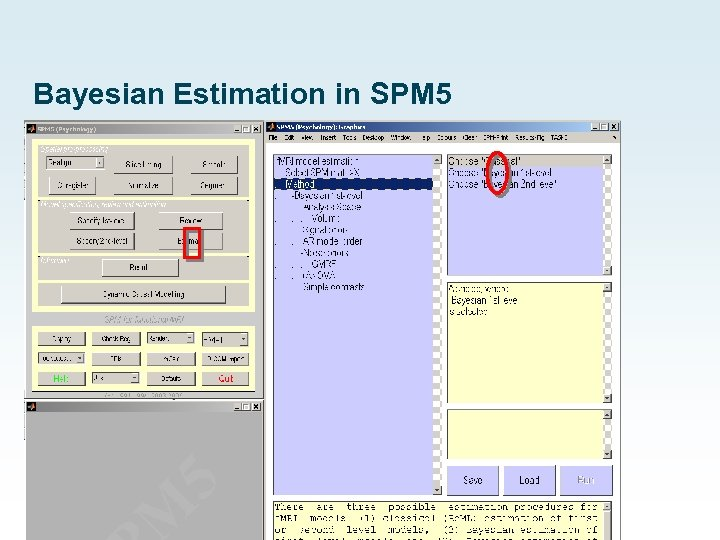Bayesian Estimation in SPM 5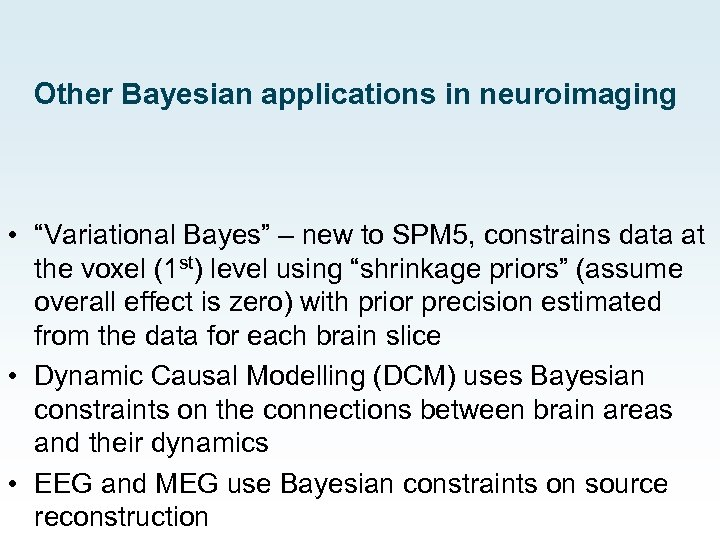Other Bayesian applications in neuroimaging • “Variational Bayes” – new to SPM 5, constrains data at the voxel (1 st) level using “shrinkage priors” (assume overall effect is zero) with prior precision estimated from the data for each brain slice • Dynamic Causal Modelling (DCM) uses Bayesian constraints on the connections between brain areas and their dynamics • EEG and MEG use Bayesian constraints on source reconstruction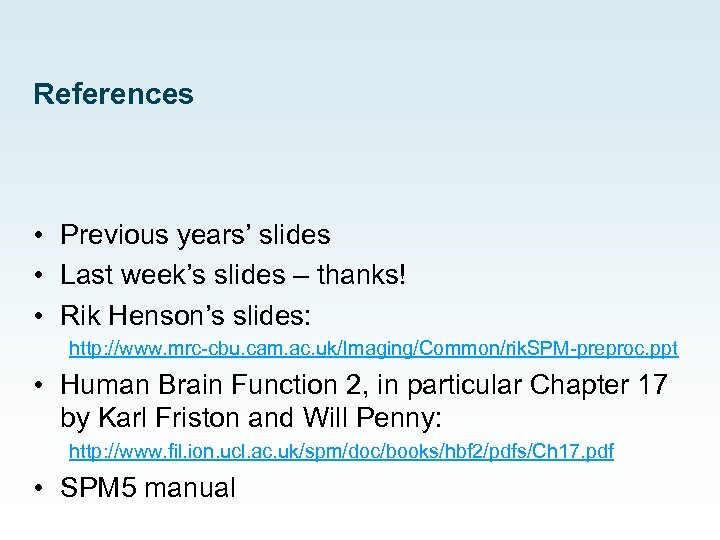References • Previous years’ slides • Last week’s slides – thanks! • Rik Henson’s slides: http: //www. mrc-cbu. cam. ac. uk/Imaging/Common/rik. SPM-preproc. ppt • Human Brain Function 2, in particular Chapter 17 by Karl Friston and Will Penny: http: //www. fil. ion. ucl. ac. uk/spm/doc/books/hbf 2/pdfs/Ch 17. pdf • SPM 5 manual